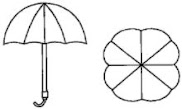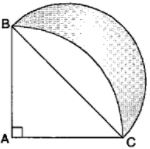# NCERT Solution for Class 10 Mathematics Chapter 12 Areas Related to Circles

 Chapter Name NCERT Solution for Class 10 Maths Chapter 12 Areas Related to Circles Topics Covered Short Revision for the ChapterNCERT Exercise Solution Related Study NCERT Solution for Class 10 MathsNCERT Revision Notes for Class 10 MathsImportant Questions for Class 10 MathsMCQ for Class 10 MathsNCERT Exemplar Questions For Class 10 Maths

## Short Revision for Areas Related to Circles

1. Perimeter of a circle = 2πr.
2. Perimeter of a semicircle = (π + 2)r.
3. Perimeter of a quadrant of a circle = (π/2 + 2)r.
4. Area of a circle = πr2 .
5. Area of a semicircle = (½)πr2 .
6. Area of a quadrant of a circle = (¼)πr2 .
7. Length of an arc of a sector of a circle with radius r and angle θ = θ/360° × 2πr.
8. Perimeter of the sector = 2r + θ/360° × 2πr.
9. Area of the sector = θ/360° × πr2 .
10. Area of a segment of a circle = area of the corresponding sector – area of the corresponding triangle.

### NCERT Exercise Solutions

#### Exercise: 12.1

1. The radii of two circles are 19 cm and 9 cm respectively. Find the radius of the circle which has a circumference equal to the sum of the circumferences of the two circles.

2. The radii of two circles are 8 cm and 6 cm, respectively. Find the radius of the circle having area equal to the sum of the areas of the two circles.

3. Fig. 12.3 depicts an archery target marked with its five scoring regions from the centre outwards as Gold, Red, Blue, Black and White. The diameter of the region representing Gold score is 21 cm and each of the other bands is 10.5 cm wide. Find the area of each of the five scoring regions.

4. The wheels of a car are of diameter 80 cm each. How many complete revolutions does each wheel make in 10 minutes when the car is travelling at a speed of 66 km per hour?

5. Tick the correct Solution: in the following and justify your choice : If the perimeter and the area of a circle are numerically equal, then the radius of the circle is
(A) 2 units
(B) π units
(C) 4 units
(D) 7 units

Solution

Option (A) is correct.

#### Exercise: 12.2

1. Find the area of a sector of a circle with radius 6 cm if angle of the sector is 60°.

2. Find the area of a quadrant of a circle whose circumference is 22 cm.

3. The length of the minute hand of a clock is 14 cm. Find the area swept by the minute hand in 5 minutes.

4. A chord of a circle of radius 10 cm subtends a right angle at the centre. Find the area of the corresponding:
(i) minor segment
(ii) major sector. (Use π = 3.14)

5. In a circle of radius 21 cm, an arc subtends an angle of 60° at the centre. Find:
(i) the length of the arc
(ii) area of the sector formed by the arc
(iii) area of the segment formed by the corresponding chord

6. A chord of a circle of radius 15 cm subtends an angle of 60° at the centre. Find the areas of the corresponding minor and major segments of the circle. (Use π = 3.14 and √3 = 1.73)

7. A chord of a circle of radius 12 cm subtends an angle of 120° at the centre. Find the area of the corresponding segment of the circle. (Use π = 3.14 and √3 = 1.73)

8. A horse is tied to a peg at one corner of a square shaped grass field of side 15 m by means of a 5 m long rope (see Fig. 12.11). Find
(i) the area of that part of the field in which the horse can graze.
(ii) the increase in the grazing area if the rope were 10 m long instead of 5 m. (Use π = 3.14)

9. A brooch is made with silver wire in the form of a circle with diameter 35 mm. The wire is also used in making 5 diameters which divide the circle into 10 equal sectors as shown in Fig. 12.12. Find:
(i) the total length of the silver wire required.
(ii) the area of each sector of the brooch.

10. An umbrella has 8 ribs which are equally spaced (see Fig. 12.13). Assuming umbrella to be a flat circle of radius 45 cm, find the area between the two consecutive ribs of the umbrella.Solution

Central angle formed by any two consecutive ribs = 360°/8 = 45°
Area between two consecutive ribs.11. A car has two wipers which do not overlap. Each wiper has a blade of length 25 cm sweeping through an angle of 115°. Find the total area cleaned at each sweep of the blades.

12. To warn ships for underwater rocks, a lighthouse spreads a red colored light over a sector of angle 80° to a distance of 16.5 km. Find the area of the sea over which the ships are warned.
(Use π = 3.14)

14. Tick the correct solution in the following:
Area of a sector of angle p (in degrees) of a circle with radius R is
(A) p/180 × 2πR
(B) p/180 × π R2
(C) p/360 × 2πR
(D) p/720 × 2πR2

#### Exercise: 12.3

1. Find the area of the shaded region in Fig. 12.19, if PQ = 24 cm, PR = 7 cm and O is the centre of the circle.

2. Find the area of the shaded region in Fig. 12.20, if radii of the two concentric circles with centre O are 7 cm and 14 cm respectively and AOC = 40°.

3. Find the area of the shaded region in Fig. 12.21, if ABCD is a square of side 14 cm and APD and BPC are semicircles.

4. Find the area of the shaded region in Fig. 12.22, where a circular arc of radius 6 cm has been drawn with vertex O of an equilateral triangle OAB of side 12 cm as centre.

Solution
= area of an equilateral triangle of side 12 cm + area of a circle of radius 6 cm - area of a sector of radius 6 cm and central angle 60°

5. From each corner of a square of side 4 cm a quadrant of a circle of radius 1 cm is cut and also a circle of diameter 2 cm is cut as shown in Fig. 12.23. Find the area of the remaining portion of the square.

6. In a circular table cover of radius 32 cm, a design is formed leaving an equilateral triangle ABC in the middle as shown in Fig. 12.24. Find the area of the design.7. In Fig. 12.25, ABCD is a square of side 14 cm. With centres A, B, C and D, four circles are drawn such that each circle touch externally two of the remaining three circles. Find the area of the shaded region.

8. Fig. 12.26 depicts a racing track whose left and right ends are semicircular.
The distance between the two inner parallel line segments is 60 m and they are each 106 m long. If the track is 10 m wide, find:
(i) the distance around the track along its inner edge
(ii) the area of the track.

9. In Fig. 12.27, AB and CD are two diameters of a circle (with centre O) perpendicular to each other and OD is the diameter of the smaller circle. If OA = 7 cm, find the area of the shaded region.

Solution
Area of the shaded region = area of circle with diameter 7 cm + area of semicircle with radius 7 cm - area of triangle with base 14 cm and height 7 cm10. The area of an equilateral triangle ABC is 17320.5 cm2. With each vertex of the triangle as centre, a circle is drawn with radius equal to half the length of the side of the triangle (see Fig. 12.28). Find the area of the shaded region. (Use π = 3.14 and √3 = 1.73205)

11. On a square handkerchief, nine circular designs each of radius 7 cm are made (see Fig. 12.29). Find the area of the remaining portion of the handkerchief.Solution

12. In Fig. 12.30, OACB is a quadrant of a circle with centre O and radius 3.5 cm. If OD = 2 cm, find the area of the

13. In Fig. 12.31, a square OABC is inscribed in a quadrant OPBQ. If OA = 20 cm, find the area of the shaded region. (Use π = 3.14)

Solution

Area of the shaded region = area of the quadrant  - area of the square14. AB and CD are respectively arcs of two concentric circles of radii 21 cm and 7 cm and centre O (see Fig. 12.32). If ∠AOB = 30°, find the area of the shaded region.

15. In Fig. 12.33, ABC is a quadrant of a circle of radius 14 cm and a semicircle is drawn with BC as diameter. Find the area of the shaded region.Solution

BC = 14√2 cm.
∴ Radius of semicircle = 7√2 cm
Area of the shaded portion = area of semicircle of radius 7√2 cm + area of an isosceles triangle with equal sides of 14 cm each - area of quadrant of radius 14 cm16. Calculate the area of the designed region in Fig. 12.34 common between the two quadrants of circles of radius 8 cm each.